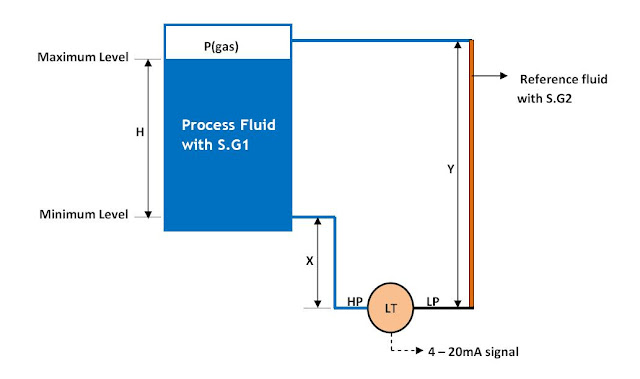Zero Elevation Calibration in Level Measurement ~ Learning Instrumentation And Control Engineering Learning Instrumentation And Control Engineering

### Zero Elevation Calibration in Level Measurement

Custom Search

Wet Leg Condition
Condensation of the gas above the process liquid slowly causes the low side of the transmitter piping to fill with liquid. The pipe is therefore purposely filled with a convenient reference fluid to eliminate this potential error. This is called a wet leg condition.

In a wet leg application, the low pressure side of the level transmitter will always experience a higher pressure than the high pressure side. This type of installation requires that the height of the wet leg will always be equal to or greater than the maximum height of the liquid column (H) inside the tank.
Consider the scenario below:Zero Elevation in Level Measurement Installation
When the liquid level is at the maximum level of height H,
P(high) = P(gas) + S.G1 * H + S.G1 * X
P(low) = P(gas) + S.G2 * Y
Differential Pressure,  = P(high) – P(low) = S.G1 * H + S.G1 * X - S.G2 * Y
 Therefore, ΔP = S.G1*(H + X) – S.G2 * Y

As can be seen, the differential pressure, ΔP, sensed by the transmitter is always a negative number (i.e. low pressure side is at a higher pressure than high pressure side) since in this application, Y is always greater than (H + X) and S.G2 is equal to or greater than S.G1. To properly calibrate this transmitter, a positive bias of (S.G2 * Y – S.G1 * X) is needed to elevate the transmitter output. This calibration technique is called Zero Elevation.
In other words, we calibrate the transmitter in the range (S.G1 * X – S.G2 * Y) to (S.G1 * H + S.G1 * X – S.G2 * Y). An example will illustrate this point:
As shown on the diagram above, if H = 550 inches, X = 60 inches, Y = 650 inches,
S.G1 = S.G2 = 1.0
Thus at full tank level of H = 550 inches,
Differential pressure sensed by the level transmitter is
= S.G1 * H + S.G1 * X - S.G2 * Y = (1.0)*(550) + (1.0)*(60) – (1.0)*(650) = -40 in wc
When tank is at the minimum level( H = 0),
Differential pressure sensed by transmitter is
= S.G1 * H + S.G1 * X - S.G2 * Y = (1.0)*(0) + (1.0)(60) – (1.0)*(650) = -590 in wc
Therefore the level transmitter should be calibrated in the range -40 in wc to -590 in wc as shown below:Zero Elevation Calibration
Want to go back to previous sections on Level Measurement?